Courses

# Batch Costing - Methods of Cost Determination, Cost Management B Com Notes | EduRev

## B Com : Batch Costing - Methods of Cost Determination, Cost Management B Com Notes | EduRev

The document Batch Costing - Methods of Cost Determination, Cost Management B Com Notes | EduRev is a part of the B Com Course Cost Management.
All you need of B Com at this link: B Com

Meaning:

Batch costing is a form of specific order costing. Job costing refers to costing of jobs that are executed against specific orders whereas in batch costing items are manufactured for stock. A finished product may require different components for assembly and may be manufactured in economical batch lots.

When orders are received from different customers, there are common products among orders; then production orders may be issued for batches, consisting of a predetermined quantity of each type of product. Batch costing method is adopted in such cases to calculate the cost of each such batch.

Cost per unit is ascertained by dividing the total cost of a batch by number of items produced in that batch. In order to do that a Batch Cost Sheet is prepared. The preparation of Batch Cost Sheet is similar to that of Job Cost Sheet. This method is mainly applied in biscuits manufacture, garments manufacture, spare parts and component manufacture, pharmaceutical enterprises etc.

Illustration 1:

Batch No. A-110 incurred the following costs:

Direct Materials -  Rs 10, 000

Department:
A - 800 labour hours @ Rs 5 per hour

B - 1400 labour hours @ Rs 6 per hour

Factory overheads are absorbed on labour hour’s basis and the rates are Rs 7 per hour for Department A and Rs 4 per hour for Department B. The firm uses a cost plus system for selling prices and expects a 25% gross profit (sales value minus factory cost). Administrative overheads are absorbed at 10% of selling price. Assuming that A-110 units were produced in Batch A-110, calculate the selling price per unit.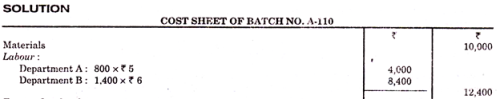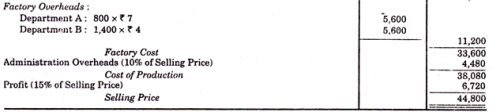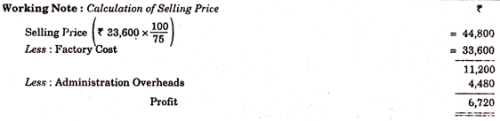Production is usually done in batches and each batch can have any number of units of Component in it. The optimum quantity for a batch is that quantity for which the setting up and carrying costs are minimum, such an optimum quantity is known as Economic Batch Quantity or Economic lot size.

Determination of the economic lot size is important in industries where batch costing is employed.

Need for Determining Economic Lot Size:

The need for determining economic lot size arises as:

(i) Every time a component/product is to be made, setting up of the tool is involved. Because of this some loss in production time will be there. Therefore, maximum number of units are produced once the machine is set in order to reduce the cost per unit,

(ii) Such large production at one run will lead to accumulation of inventory and the costs related thereto,

(iii) Thus there is a quantity for which reduced cost of production is just offset by costs of carrying the quantity inventory. The determination of most economical batch quantity requires consideration of many related factors of costs and economies.

The factors that influence the decision in this respect are:

(a) Set up cost,

(b) Manufacturing cost,

(c) Interest on capital,

(iv) Storage cost, and

(v) Rate of consumption.

Types of Costs in Batch Costing:

There are two types of costs involved in Batch Costing:

(i) Set up costs

(ii) Carrying costs.

If the batch size is increased, set up cost per unit will come down and the carrying cost will increase. If the batch size is reduced, set up cost per unit will increase and the carrying cost will come down. Economic Batch quantity will balance both these opponent costs.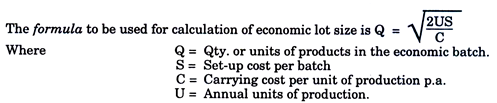Illustration 2:

Compute the economic batch quantity for a company using batch costing with the following information: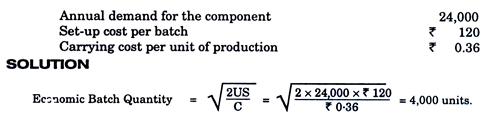Illustration 3:

The annual demand of a product is 24,000 units. It is produced in batches and the largest size of a single batch is 6,000 units. After each batch is complete, the set up cost is Rs. 750. The annual carrying cost is Rs. 2.25 per unit.

Assume average inventory as one-half of the number of units made in each batch . Selecting 4, 6, 8, 12 and 24 batches per annum, determine annual costs of each and state the optimum number of batches to minimize the total costs.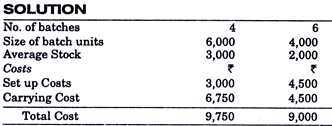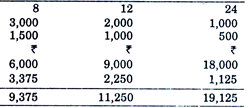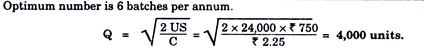Illustration 4:

The demand of an item is uniform at a rate of 25 units p.m. The set up cost is Rs. 30 each time a production is made. The production cost is Rs. 3 per item and the inventory carrying cost is 50 paise per unit p.m. If the shortage cist is Rs. 3 per item p.m. determine how often to make a production run and of what size? Also calculate re-order level.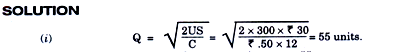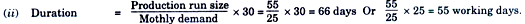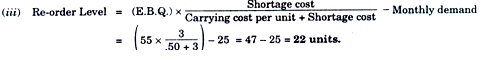Difference Between Job Costing and Batch Costing:

In case of job costing, work is undertaken as an identifiable unit and cost of each job is ascertained separately. Such a method of costing is suitable in case of motor workshop, printing press and where manufacture of products is according to customers’ specific requirements.

Batch costing is extension of job costing. Job costing refers to costing of jobs that are executed against specific orders whereas in batch costing items are manufactured for stock. In batch costing a batch may represent a number of small orders passed through the factory in batches. Each batch is treated as a unit of cost and is separately costed. Cost per unit is ascertained by dividing the total cost of the batch by number of items produced in that batch.

Offer running on EduRev: Apply code STAYHOME200 to get INR 200 off on our premium plan EduRev Infinity!

## Cost Management

52 videos|51 docs

,

,

,

,

,

,

,

,

,

,

,

,

,

,

,

,

,

,

,

,

,

,

,

,

;